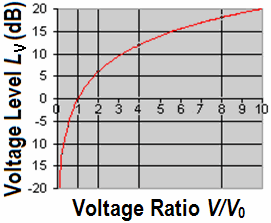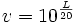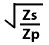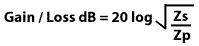Deutsche VersionAmplifier  Conversion - Gain / Loss

Gain means signal strength (amplitude) or amplification.
In audio we use only voltage amplification. Forget the power.

The amplification factor, also called gain, is the extent to which an active device boosts the strength of a signal.
The damping factor, also called loss, is the extent to which a passive device reduces the strength of a signal.
Amplification means voltage ratio
V2 / V1 = Vout / Vin, and voltage gain in dB = 20 × log (V2 / V1).
 Gain in decibel (+dB) Loss in decibel (−dB) Voltage Ratio Gain Voltage Ratio Loss

● Convert Voltage Gain / Loss to Decibels ●

 Voltage Ratio Gain Voltage Ratio Loss Gain in decibel (+dB) Loss in decibel (−dB)

• Voltage and Gain •

 Enter any two values - the third will be calculated.

 Reference voltage V0 µV mV V Measured voltage V µV mV V Gain G or LV dBV0 = 1 Volt

Amplification and Damping

 To use the calculator, simply enter a value. The calculator works in both directions of the ↔ sign.

 Gain factor v = V2 ⁄ V1 (ratio) for field sizes, e.g. voltage ↔ Amplification level L   voltage level dB0 dB = voltage gain v = 1

Gain and Loss

 Gain is: 1) A knob usually found at the top of each input channel on the sound board, used to set input levels of the separate channels to relatively equal positions. 2) The amount of increase in audio signal strength, often expressed in dB. Gain:The measure of how much a circuit amplifies a signal. Gain may be stated as a ratio of input to output voltage,current or power, such as a voltage gain of 4, or a power gain of 2, or it can be expressed in decibels, such as a line amplifier with a gain of 10 dB. Conductive Hearing Loss: a loss of sensitivity to sound, resulting from an abnormality or blockage of the outer ear or the middle ear. The most common cause of conductive hearing loss is middle ear fluid or infection. Other causes include wax build-up in the ear canal, a hole in the eardrum, or damage to the tiny bones of the middle ear. Gain or Loss: These are reciprocal numbers; they will be given in several ways. Usually it is expressed as a ratio. It can be stated as ratio of the primary to secondary impedances of an audio transformer. This is given by gain or loss =This voltage can also be expressed in dB as well. It is given by the formula:also voltages across the primary and secondary. dB (decibel): A technique for expressing voltage,power, gain, loss, or frequency in logarithmic form against a reference.Typical references include volts, watts or Hz. DeciBels are calculated using the expression: Level in decibel L = 20 × log (x/y) or L = 10 · log (x/y). Voltage Controlled Attenuator (VCA): Similar to a voltage controlled amplifier except that the amplifier will, with no control voltage sent to it, have no gain and no loss; as an increasing control voltage is sent to it, the amplifier reduces gain, causing an amplitude loss of signal strength.

 Sound level, loudness, and sound pressure are not the same things. There are variations in individual perception of the strength of sound. The sound pressure measured twice as much gives 6 dB more level. The sound perceived twice as loud needs roughly an increase in sound level by 10 dB.

Common Gains and Losses Expressed in Decibels
Notice: Power gain is not used in audio engineering.

 Gain/loss      as a ratio (out/in) Gain/loss      as a factor Gain/loss in dB     Field sizes   (Voltage gain) Gain/loss in dB   Energy sizes (Power gain) 10,000:1 10,000 +80.00 dB +40.00 dB 1,000:1 1,000 +60.00 dB +30.00 dB 100:1 100 +40.00 dB +20.00 dB 10:1 10 +20.00 dB +10.00 dB 5:1 5 +13.98 dB +6.99 dB 4:1 4 +12.04 dB +6.02 dB ● 2:1 2 +6.02 dB ● +3.01 dB 1:1 1 0,00 dB 0.00 dB 1:2 0.5 −6.02 dB ● −3.01 dB 1:4 0.25 −12.04 dB −6.02 dB ● 1:5 0.2 −13.98 dB −6.99 dB 1:10 0.1 −20.00 dB −10.00 dB 1:100 0.01 −40.00 dB −20.00 dB 1:1,000 0.001 −60.00 dB −30.00 dB 1:10,000 0.0001 −80.00 dB −40.00 dB

40 dB voltage gain (amplitude) is 100 times the voltage factor (amplitude).
40 dB power gain is 10,000 times the power factor.

Voltage Gain = Voltage Amplification and Power Gain = Power amplification

 Voltage (amplitude) gain Power (energy) gain Voltage ratio Factor V2/V1 Voltage amplification GV in dB Power ratio Factor P2/P1 Power amplification GP in dB 103 +60 106 +60 102 +40 104 +40 101 +20 102 +20 √10 = 3.16 +10 10 +10 2 +6 4 +6 √2 = 1.414 +3 2 +3 1 ±0 1 ±0 1/√2 = 0.7071 −3 1/2 = 0.5 −3 1/2 = 0.5 −6 1/4 = 0.25 −6 1/√10 = 0.316 −10 10−1 = 0.1 −10 10−1 = 0.1 −20 10−2 = 0.01 −20 10−2 = 0.01 −40 10−4 = 0.0001 −40 10−3 = 0.001 −60 10−6 = 0.000001 −60 V2/V1 = 10(GV in dB/20) GV = 20×log (V2/V1) P2/P1 = 10(GP in dB/10) GP = 10×log (P2/P1)The word "power amplifier" is a misnomer - especially in audio engineering. Voltage and current can be amplified. The strange term "power amplifier" has become understood to mean an amplifier that is intended to drive a load such as a loudspeaker. We call the product of current and voltage gain "power amplification".

 Questions: For an amplifier with an amplification factor of 100, calculate the following: a) voltage gain in dB. b) voltage gain at the cutoff frequency (break frequency) in dB. c) amplification factor at the cutoff frequency (break frequency). Answers: a) amplification factor 100 = gain 40 dB b) gain at the cutoff frequency is 3 dB, so it is 37 dB. c) gain 37 dB = amplification factor 70.7 at the cutoff frequency.